# Prime element

## Definition

### Symbol-free definition

A nonzero element in an integral domain is said to be a prime element if it satisfies the following equivalent conditions:

### Definition with symbols

A nonzero element$p$ in an integral domain$R$ is said to be prime' if it satisfies the following:

• Whenever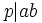$p|ab$, then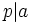$p|a$ or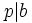$p|b$.
• The principal ideal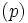$(p)$ is a prime ideal in$R$, or equivalently,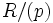$R/(p)$ is an integral domain.

### Invariance up to associates

Further information: Prime element property is invariant upto associates

Given two associate elements, one of them is prime if and only if the other one is.